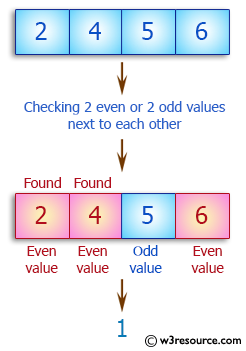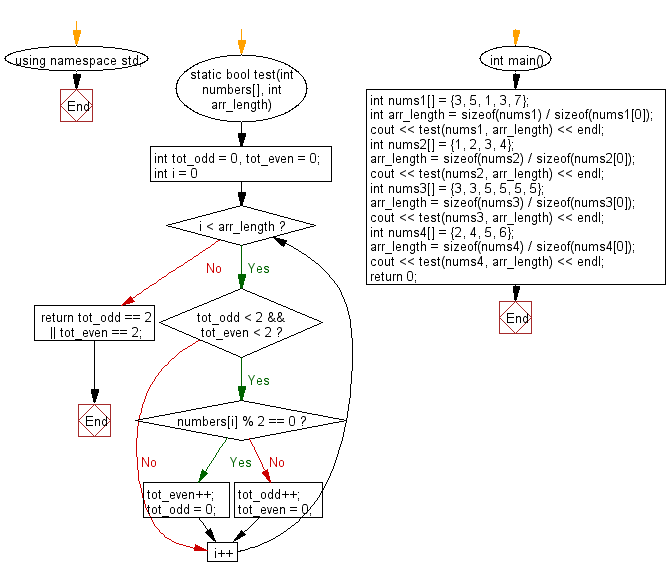﻿ C++ Exercises: Check a given array of integers and return true if the given array contains either 2 even or 2 odd values all next to each other - w3resource# C++ Exercises: Check a given array of integers and return true if the given array contains either 2 even or 2 odd values all next to each other

## C++ Basic Algorithm: Exercise-111 with Solution

Write a C++ program to check a given array of integers and return true if the given array contains either 2 even or 2 odd values all next to each other.

Sample Solution:

C++ Code :

``````#include <iostream>
using namespace std;

static bool test(int numbers[], int arr_length)
{
int tot_odd = 0, tot_even = 0;

for (int i = 0; i < arr_length; i++)
{
if (tot_odd < 2 && tot_even < 2)
{
if (numbers[i] % 2 == 0)
{
tot_even++;
tot_odd = 0;
}
else
{
tot_odd++;
tot_even = 0;
}
}
}
return tot_odd == 2 || tot_even == 2;
}

int main()
{
int nums1[] = {3, 5, 1, 3, 7};
int arr_length = sizeof(nums1) / sizeof(nums1);
cout << test(nums1, arr_length) << endl;
int nums2[] = {1, 2, 3, 4};
arr_length = sizeof(nums2) / sizeof(nums2);
cout << test(nums2, arr_length) << endl;
int nums3[] = {3, 3, 5, 5, 5, 5};
arr_length = sizeof(nums3) / sizeof(nums3);
cout << test(nums3, arr_length) << endl;
int nums4[] = {2, 4, 5, 6};
arr_length = sizeof(nums4) / sizeof(nums4);
cout << test(nums4, arr_length) << endl;
return 0;
}
``````

Sample Output:

```1
0
1
1
```

Pictorial Presentation:Flowchart:C++ Code Editor:

Contribute your code and comments through Disqus.

What is the difficulty level of this exercise?

﻿